• 滑动窗口 滑动窗口就是能够根据指定的单位长度来框住时间序列，从而计算框内的统计指标。相当于一个长度指定的滑块正在刻度尺上面滑动，每滑动一个单位即可反馈滑块内的数据。... 移动窗口就是窗口向一端滑行，每次时间序列 滑动窗口
• 针对双目基于ORB特征的即时定位与地图构建（ORB-SLAM）算法位姿估计精度较低的问题，对其进行了改进，提出了一种基于滑窗非线性优化的双目视觉SLAM算法。该算法引入了滑窗思想，在状态估计过程中对最新多帧图像对应...
•机器学习
• 3*3的窗口滑动，基于verilog语言写成的，和源码找好了，打包在一起用8个积分吸引有缘人，哈哈，如果你也要用FPGA做数字图像处理，我觉得你一定会选择这个资源。verilog
• 针对低检测概率下的无源定位问题,提出一种基于滑窗批处理的多传感器融合跟踪算法。通过将多个低检测概率的无源传感器组网,实现目标信息的空间积累,有效提高目标的检测概率。利用伪线性估计技术将非线性测量转换为伪...
• 检测中非线性负载产生的谐波电流成分，运算过程中应用了滑窗迭代算法，它能有效地提高系统的实时性、目标跟随特性和抗干扰性，并且具有计算量小、容易工程实现的特点。进行了滑窗迭代算法的Matlab仿真试验，结果表明...
• 针对现有检测方法的不足,提出一种基于离散傅里叶变换的滑窗迭代DFT算法,实时测出谐波参考指令电流。该算法计算量小、容易工程实现,经过扩展,该算法还能应用于检测特定谐波。仿真实验结果验证了该算法的有效性和可行...
• 讨论滑窗法航迹起始的理论及其应用．并从实际系统的大量统计中得出仅用滑窗法很难解决航迹快速起始的能力与高虚假航迹概率之间的矛盾。
•研究论文
• * 该滑窗的起始创建时间，也就是第一个数据 */ private long beginTimestamp; /** * 最后一个数据的时间戳 */ private long lastAddTimestamp; public static void main(String[] args) { //1秒一个时间片...
由于最近有一个统计单位时间内某key的访问次数的需求，譬如每5秒访问了redis的某key超过100次，就取出该key单独处理。
这样的单位时间统计，很明显我们都知道有个边界问题，譬如5秒内100次的限制。刚好前4.99秒访问都是0，最后0.01秒来了100次，5.01秒又来了100次。也就是访问有明显的毛刺情况出现，为了弱化这个毛刺情况，我们可以采用滑动窗口。
滑动窗口
滑动窗口的主要原理比较简单，就是将这个单位时间进行拆分，譬如5秒的统计范围，我们将它划分成5个1秒。
当请求进来时，先判断当前请求属于这5个1秒的时间片中的哪个，然后将对应的时间片对应的统计值加1，再判断当前加上前4个时间片的次数总和是否已经超过了设置的阈值。
当时间已经到达第6个时间片时，就把第一个时间片给干掉，因为无论第一片是多少个统计值，它都不会再参与后续的计算了。
就这样，随着时间的推移，统计值就随着各个时间片的滚动，不断地进行统计。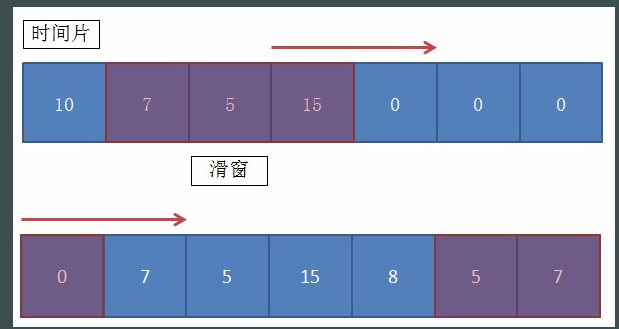具体要将单位时间拆分为多少片，要根据实际情况来决定。当然，毫无疑问的是切分的越小，毛刺现象也越少。系统统计也越准确，随之就是内存占用会越大，因为你的这个窗口的数组会更大。
代码实现思路就是定义好分片数量，每个分片都有一个独立的计数器，所有的分片合计为一个数组。当请求来时，按照分片规则，判断请求应该划分到哪个分片中去。要判断是否超过阈值，就将前N个统计值相加，对比定义的阈值即可。
代码我直接引用别人写好的了，源代码在https://www.iteye.com/blog/go12345-1744728

import java.util.concurrent.atomic.AtomicInteger;

/**
* 滑动窗口。该窗口同样的key，都是单线程计算。
*
* @author wuweifeng wrote on 2019-12-04.
*/
public class SlidingWindow {
/**
* 循环队列，就是装多个窗口用，该数量是windowSize的2倍
*/
private AtomicInteger[] timeSlices;
/**
* 队列的总长度
*/
private int timeSliceSize;
/**
* 每个时间片的时长，以毫秒为单位
*/
private int timeMillisPerSlice;
/**
* 共有多少个时间片（即窗口长度）
*/
private int windowSize;
/**
* 在一个完整窗口期内允许通过的最大阈值
*/
private int threshold;
/**
* 该滑窗的起始创建时间，也就是第一个数据
*/
private long beginTimestamp;
/**
* 最后一个数据的时间戳
*/

public static void main(String[] args) {
//1秒一个时间片，窗口共5个
SlidingWindow window = new SlidingWindow(100, 4, 8);
for (int i = 0; i < 100; i++) {

window.print();
System.out.println("--------------------------");
try {
} catch (InterruptedException e) {
e.printStackTrace();
}
}
}

public SlidingWindow(int duration, int threshold) {
//超过10分钟的按10分钟
if (duration > 600) {
duration = 600;
}
//要求5秒内探测出来的，
if (duration <= 5) {
this.windowSize = 5;
this.timeMillisPerSlice = duration * 200;
} else {
this.windowSize = 10;
this.timeMillisPerSlice = duration * 100;
}
this.threshold = threshold;
// 保证存储在至少两个window
this.timeSliceSize = windowSize * 2;

reset();
}

public SlidingWindow(int timeMillisPerSlice, int windowSize, int threshold) {
this.timeMillisPerSlice = timeMillisPerSlice;
this.windowSize = windowSize;
this.threshold = threshold;
// 保证存储在至少两个window
this.timeSliceSize = windowSize * 2;

reset();
}

/**
* 初始化
*/
private void reset() {
beginTimestamp = SystemClock.now();
//窗口个数
AtomicInteger[] localTimeSlices = new AtomicInteger[timeSliceSize];
for (int i = 0; i < timeSliceSize; i++) {
localTimeSlices[i] = new AtomicInteger(0);
}
timeSlices = localTimeSlices;
}

private void print() {
for (AtomicInteger integer : timeSlices) {
System.out.print(integer + "-");
}
}

/**
* 计算当前所在的时间片的位置
*/
private int locationIndex() {
long now = SystemClock.now();
//如果当前的key已经超出一整个时间片了，那么就直接初始化就行了，不用去计算了
if (now - lastAddTimestamp > timeMillisPerSlice * windowSize) {
reset();
}

return (int) (((now - beginTimestamp) / timeMillisPerSlice) % timeSliceSize);
}

/**
* 增加count个数量
*/
//当前自己所在的位置，是哪个小时间窗
int index = locationIndex();
//        System.out.println("index:" + index);
//然后清空自己前面windowSize到2*windowSize之间的数据格的数据
//譬如1秒分4个窗口，那么数组共计8个窗口
//当前index为5时，就清空6、7、8、1。然后把2、3、4、5的加起来就是该窗口内的总和
clearFromIndex(index);

int sum = 0;
// 在当前时间片里继续+1
//加上前面几个时间片
for (int i = 1; i < windowSize; i++) {
sum += timeSlices[(index - i + timeSliceSize) % timeSliceSize].get();
}
System.out.println(sum + "---" + threshold);

return sum >= threshold;
}

private void clearFromIndex(int index) {
for (int i = 1; i <= windowSize; i++) {
int j = index + i;
if (j >= windowSize * 2) {
j -= windowSize * 2;
}
timeSlices[j].set(0);
}
}

}

import java.util.concurrent.Executors;
import java.util.concurrent.ScheduledExecutorService;
import java.util.concurrent.TimeUnit;
import java.util.concurrent.atomic.AtomicLong;

/**
* 用于解决高并发下System.currentTimeMillis卡顿
* @author lry
*/
public class SystemClock {

private final int period;

private final AtomicLong now;

private static class InstanceHolder {
private static final SystemClock INSTANCE = new SystemClock(1);
}

private SystemClock(int period) {
this.period = period;
this.now = new AtomicLong(System.currentTimeMillis());
scheduleClockUpdating();
}

private static SystemClock instance() {
return InstanceHolder.INSTANCE;
}

private void scheduleClockUpdating() {
@Override
}
});
scheduler.scheduleAtFixedRate(() -> now.set(System.currentTimeMillis()), period, period, TimeUnit.MILLISECONDS);
}

private long currentTimeMillis() {
return now.get();
}

/**
* 用来替换原来的System.currentTimeMillis()
*/
public static long now() {
return instance().currentTimeMillis();
}
}

参照代码main方法，通过修改每个时间片的时间，窗口数量，阈值，来进行测试。
这就是简单实现了。


展开全文滑动窗口 java滑动窗口
• 滑动窗口的实现到底有多难，今天在做课程设计的时候，无意中实现了。。。
• 为了满足煤矿变电站供电系统实时准确、综合动态补偿谐波的要求,提出了一种基于坐标变换的离散傅里叶变换滑窗迭代电力谐波检测算法。该算法能够有效准确地检测出谐波参考指令电流,提高了系统的实时性、抗干扰性及检测...
• 在vs2010中实现滑动窗口的简单模拟，程序有不严谨的地方，只是一个简单的实现模拟功能滑动窗口模拟
• 改进型滑窗DFT算法在静止无功发生器中的应用，张昆，，电网中大容量感性或容性设备的应用，使得电网中无功功率增大，线路中无功损耗增加，严重影响着工业生产的正常运行。静止无功发生
• 通过卫星影像进行船只检测 ...船舶检测器将一个窗口过图像金字塔，并将每个窗口分类为船舶还是非船舶，并返回一组边界框，这些边界框随后使用非最大压缩方案进行过滤。 python ship_detector.pyPython
• ## 滑窗法

千次阅读 2019-10-15 09:58:58
1、 滑窗法的内涵： （1）思想：滑动串口。 （2）格式：lambda 参数 ：返回值，其中参数可以是一个也可以是多个。 （2）注意：lambda 并不会带来程序运行效率的提高，只会使代码更简洁。 2、例子说明 （1）求两...
1、 滑窗法的内涵：
（1）思想：滑动窗口在字符串或者数组移动，直到末尾。 （2）作用：降低for循环嵌套的深度，通常情况下时间复杂度为O(N) （3）注意：滑动窗口的长度可以动态变化，需要两个指针分别指向窗口的左边界和右边界。
2、例子说明
（1）求数组中连续k数之和的最大值   方法一：暴力法（时间复杂度O(N2))
def getMaxSumkElements2(list, k):
"""
list:python中为列表类型
k:整数,默认k<=len(list)
"""
size = len(list)
maxsum = 0
for i in range(size-k+1):  # 两层for循环嵌套，复杂度高
sum = 0
for j in range(i, i+k):
sum += list[j]
maxsum = max(maxsum, sum)
return maxsum

a = [-2,1,-3,4,-1,2,1,-5,4]
print(getMaxSumkElements2(a, 4))
6

方法2：滑窗法（时间复杂度O(N)）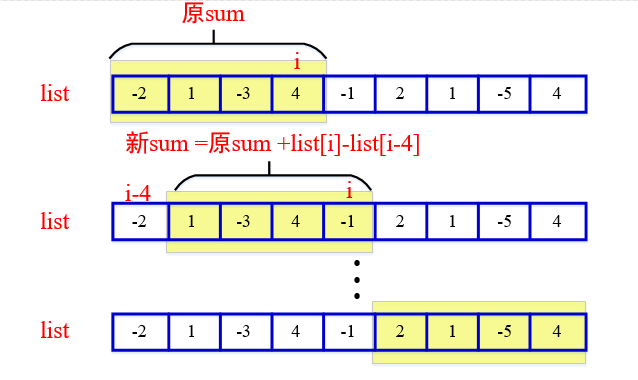def getMaxSumkElements(list, k):
"""
list:python中为列表类型
k:整数,默认k<=len(list)
"""
size = len(list)
sum = 0
maxsum = 0
for i in range(0, size):  # 算法时间复杂度O(N)
if i < k:
sum += list[i]
else:
sum = sum + list[i] - list[i-k]  # 核心代码
maxsum = max(maxsum, sum)
return maxsum

a = [-2,1,-3,4,-1,2,1,-5,4]
print(getMaxSumkElements(a, 4))
6

（2）无重复子串的最长子串def lengthOfLongestSubstring(s):
position = {} # 字符和它的邻接索引的键值对
pre = 0  # pre窗口左界,i窗口右边界
maxlength = 0 # 最大子串长度
for i in range(len(s)):
if s[i] in position: # 如果和窗口内的重复
pre = max(pre, position[s[i]])  # 窗口左界更新
maxlength = max(maxlength, i - pre + 1)  # 求最大子串
position[s[i]] = i + 1  #
return maxlength  # 建立字符和它的邻接索引的键值对

s = "pwwkew"
print(lengthOfLongestSubstring(s))
ans:3

展开全文滑动窗口法
• 用于分类高分辨率全幻灯片图像的滑动框架，通常是显微镜或组织病理学图像
• 高速信号采集的滑窗检测，童浩然，，本文给出了一种在高速信号采集系统中基于滑窗检测的回波检测方案，该算法不仅能在噪声背景下检测出信号，而且能准确捕获雷达回波SAR
• 滑窗法在算法题中大量应用，其思想简洁强大，但是往往在维护左右指针时候容易出错，现总结整理如下：
滑窗法在算法题中大量应用，其思想简洁强大，但是往往在维护左右指针时候容易出错，现总结整理如下：
1. 介绍
滑动窗口法，也叫尺取法（可能也不一定相等，大概就是这样 =。=），可以用来解决一些查找满足一定条件的连续区间的性质（长度等）的问题。由于区间连续，因此当区间发生变化时，可以通过旧有的计算结果对搜索空间进行剪枝，这样便减少了重复计算，降低了时间复杂度。往往类似于“请找到满足xx的最x的区间（子串、子数组）的xx”这类问题都可以使用该方法进行解决。
2. 引入的小例子
2.1 Leetcode 209. 长度最小的子数组
给定一个含有 n 个正整数的数组和一个正整数 s ，找出该数组中满足其和 ≥ s 的长度最小的连续子数组。如果不存在符合条件的连续子数组，返回 0。

示例:

输入: s = 7, nums = [2,3,1,2,4,3]
输出: 2
解释: 子数组 [4,3] 是该条件下的长度最小的连续子数组。

这道题目最简单的解法自然是枚举每个数组起点和终点，这种解法的时间复杂度是O(N^2)
def solver(nums, s):
optim = len(nums) + 1
for start in range(len(nums)):
summation = 0
for end in range(start, len(nums)):
summation += nums[end]
if summation >= s:
optim = min(optim, end - start + 1)
break
return optim

3. 滑动窗口法的大体框架
滑动窗口算法的思路是这样：
1、我们在字符串 S 中使用双指针中的左右指针技巧，初始化 left = right = 0，把索引闭区间 [left, right] 称为一个「窗口」。
2、我们先不断地增加 right 指针扩大窗口 [left, right]，直到窗口中的字符串符合要求（包含了 T 中的所有字符）。
3、此时，我们停止增加 right，转而不断增加 left 指针缩小窗口 [left, right]，直到窗口中的字符串不再符合要求（不包含 T 中的所有字符了）。同时，每次增加 left，我们都要更新一轮结果。
4、重复第 2 和第 3 步，直到 right 到达字符串 S 的尽头。
这个思路其实也不难，第 2 步相当于在寻找一个「可行解」，然后第 3 步在优化这个「可行解」，最终找到最优解。左右指针轮流前进，窗口大小增增减减，窗口不断向右滑动。
下面画图理解一下，needs 和 window 相当于计数器，分别记录 T 中字符出现次数和窗口中的相应字符的出现次数。
初始状态：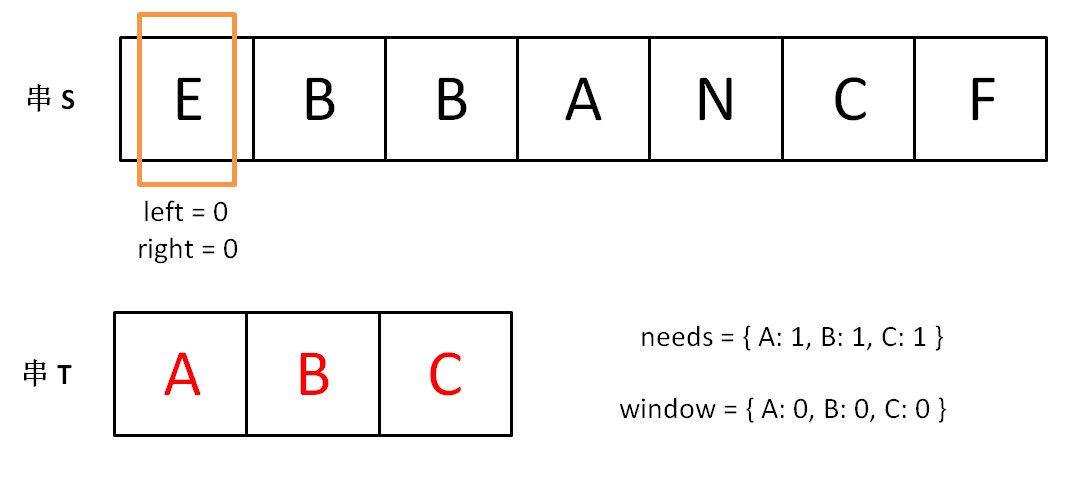增加 right，直到窗口 [left, right] 包含了 T 中所有字符：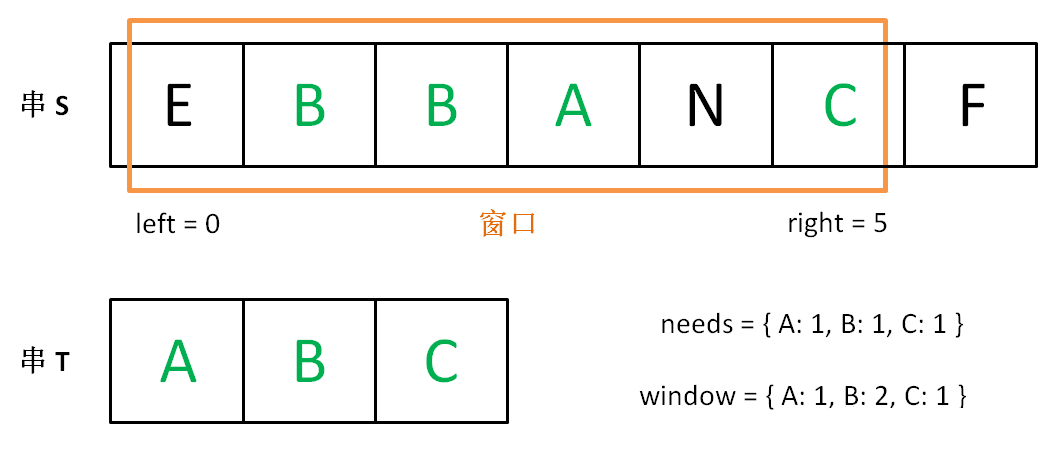现在开始增加 left，缩小窗口 [left, right]。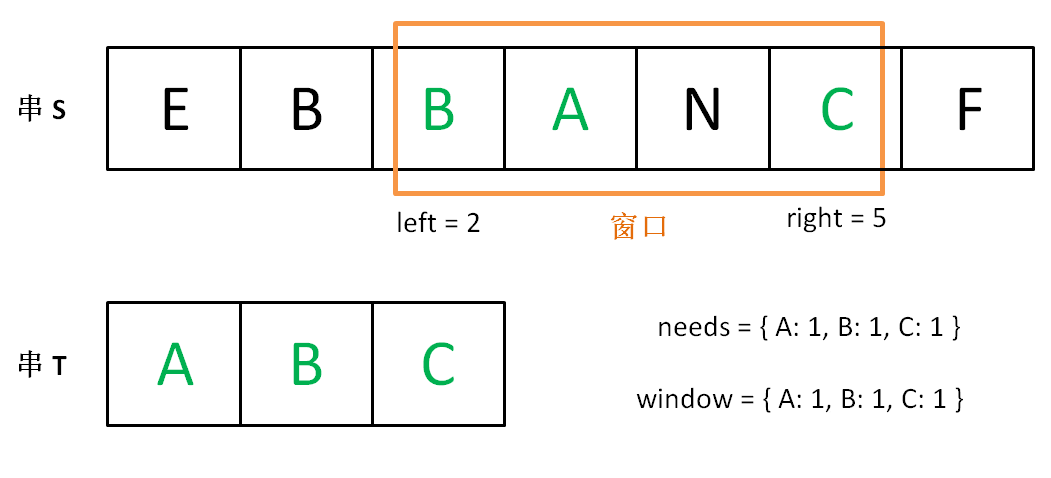直到窗口中的字符串不再符合要求，left 不再继续移动。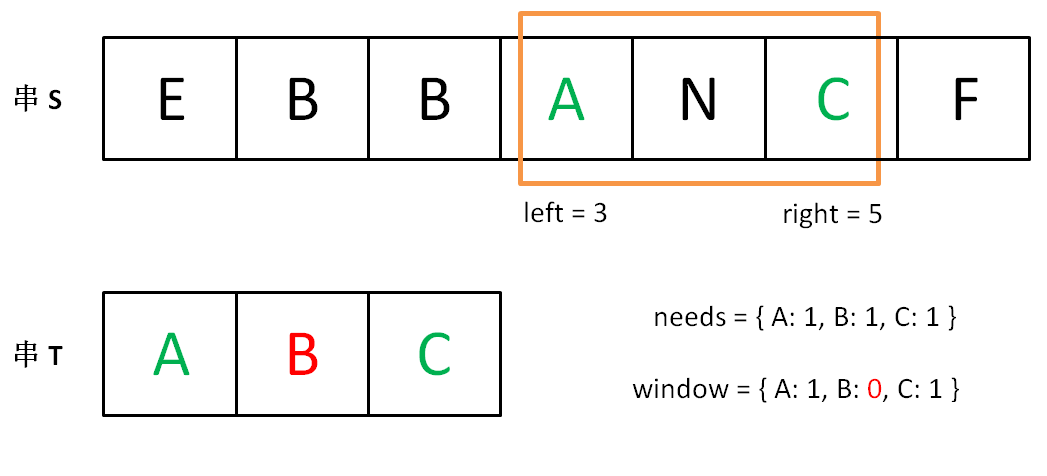之后重复上述过程，先移动 right，再移动 left…… 直到 right 指针到达字符串 S 的末端，算法结束。
如果你能够理解上述过程，恭喜，你已经完全掌握了滑动窗口算法思想。至于如何具体到问题，如何得出此题的答案，都是编程问题，等会提供一套模板，理解一下就会了。
上述过程可以简单地写出如下伪码框架：
	string s, t;
// 在 s 中寻找 t 的「最小覆盖子串」
int left = 0, right = 0;
string res = s;

while(right < s.size()) {
right++;
// 如果符合要求，移动 left 缩小窗口
while (window 符合要求) {
// 如果这个窗口的子串更短，则更新 res
res = minLen(res, window);
window.remove(s[left]);
left++;
}
}
return res;


如果上述代码你也能够理解，那么你离解题更近了一步。关注我的众公号 labuladong 看更多精彩算法文章。现在就剩下一个比较棘手的问题：如何判断 window 即子串 s[left…right] 是否符合要求，是否包含 t 的所有字符呢？
可以用两个哈希表当作计数器解决。用一个哈希表 needs 记录字符串 t 中包含的字符及出现次数，用另一个哈希表 window 记录当前「窗口」中包含的字符及出现的次数，如果 window 包含所有 needs 中的键，且这些键对应的值都大于等于 needs 中的值，那么就可以知道当前「窗口」符合要求了，可以开始移动 left 指针了。
现在将上面的框架继续细化：
	string s, t;
// 在 s 中寻找 t 的「最小覆盖子串」
int left = 0, right = 0;
string res = s;

// 相当于两个计数器
unordered_map<char, int> window;
unordered_map<char, int> needs;
for (char c : t) needs[c]++;

// 记录 window 中已经有多少字符符合要求了
int match = 0;

while (right < s.size()) {
char c1 = s[right];
if (needs.count(c1)) {
window[c1]++; // 加入 window
if (window[c1] == needs[c1])
// 字符 c1 的出现次数符合要求了
match++;
}
right++;

// window 中的字符串已符合 needs 的要求了
while (match == needs.size()) {
// 更新结果 res
res = minLen(res, window);
char c2 = s[left];
if (needs.count(c2)) {
window[c2]--; // 移出 window
if (window[c2] < needs[c2])
// 字符 c2 出现次数不再符合要求
match--;
}
left++;
}

}
return res;

上述代码已经具备完整的逻辑了，只有一处伪码，即更新 res 的地方，不过这个问题太好解决了，直接看解法吧！
string minWindow(string s, string t) {
// 记录最短子串的开始位置和长度
int start = 0, minLen = INT_MAX;
int left = 0, right = 0;

unordered_map<char, int> window;
unordered_map<char, int> needs;
for (char c : t) needs[c]++;

int match = 0;

while (right < s.size()) {
char c1 = s[right];
if (needs.count(c1)) {
window[c1]++;
if (window[c1] == needs[c1])
match++;
}
right++;

while (match == needs.size()) {
if (right - left < minLen) {
// 更新最小子串的位置和长度
start = left;
minLen = right - left;
}
char c2 = s[left];
if (needs.count(c2)) {
window[c2]--;
if (window[c2] < needs[c2])
match--;
}
left++;
}
}
return minLen == INT_MAX ?
"" : s.substr(start, minLen);
}


如果直接甩给你这么一大段代码，我想你的心态是爆炸的，但是通过之前的步步跟进，你是否能够理解这个算法的内在逻辑呢？你是否能清晰看出该算法的结构呢？
这个算法的时间复杂度是 O(M + N)，M 和 N 分别是字符串 S 和 T 的长度。因为我们先用 for 循环遍历了字符串 T 来初始化 needs，时间 O(N)，之后的两个 while 循环最多执行 2M 次，时间 O(M)。
读者也许认为嵌套的 while 循环复杂度应该是平方级，但是你这样想，while 执行的次数就是双指针 left 和 right 走的总路程，最多是 2M 嘛。
4. 滑动窗口法实例
4.1. LeetCode 76. 最小覆盖子串
给你一个字符串 S、一个字符串 T，请在字符串 S 里面找出：包含 T 所有字母的最小子串。
示例：
输入: S = "ADOBECODEBANC", T = "ABC"
输出: "BANC"

说明：
如果 S 中不存这样的子串，则返回空字符串 “”。如果 S 中存在这样的子串，我们保证它是唯一的答案。
思路1：
class Solution {
public:
string minWindow(string s, string t) {
unordered_map<char, int> window;
unordered_map<char, int> target;
for (char c : t) target[c]++;

int left = 0, right = 0;
int windowCnt = 0;
int length = s.size();
int start = 0, minLen = INT_MAX;
while (right < length) {
if (target.count(s[right])) {
window[s[right]]++;
if (window[s[right]] == target[s[right]]) {
windowCnt++;
}
}
right ++;
while (windowCnt == target.size()) {
if (right - left < minLen) {
start = left;
minLen = right - left;
}
char temp = s[left];
if (target.count(temp)) {
window[temp]--;
if (window[temp] < target[temp]) {
windowCnt--;
}
}
left ++;
}
}
return minLen == INT_MAX? "": s.substr(start, minLen);
}
};

4.2 Leetcode 209. 长度最小的子数组
给定一个含有 n 个正整数的数组和一个正整数 s ，找出该数组中满足其和 ≥ s 的长度最小的连续子数组。如果不存在符合条件的连续子数组，返回 0。

示例:

输入: s = 7, nums = [2,3,1,2,4,3]
输出: 2
解释: 子数组 [4,3] 是该条件下的长度最小的连续子数组。

这道题是上面讲解过的题目，这里套用之前提出的框架再讲一遍。我们设置一个状态为summation，表示当前区间的和，而状态满足的条件是summation >= s，寻找最优值则是去比较当前的最优值以及目前滑动窗口的长度。代入框架即得到了求解该问题的程序：
def minSubArrayLen(s: int, nums: List[int]) -> int:
summation = 0
L, R = 0, -1
optim = len(nums) + 1
while R < len(nums):
while R < len(nums):
R += 1
if R < len(nums):
summation += nums[R]
if summation >= s:
optim = min(optim, R - L + 1)
break

if R == len(nums):
break

while L < R:
summation -= nums[L]
L += 1
if summation >= s:
optim = min(optim, R - L + 1)
else:
break
return optim if optim != len(nums) + 1 else 0

方法2：
class Solution:
def minSubArrayLen(self, s: int, nums: List[int]) -> int:
if not nums:
return 0
left = right = 0
temp_res = 0
res = len(nums) + 1
while right < len(nums):
temp_res += nums[right]
while temp_res >= s:
res = min(res, right - left + 1)
temp_res -= nums[left]
left += 1
right += 1
return res if res != len(nums) + 1 else 0

内部只用一个 while 循环，用于维护滑窗满足要求，不容易出错，是推荐解题思路，模板做法容易出错！
4.3 Leetcode 3. 无重复字符的最长子串
给定一个字符串，请你找出其中不含有重复字符的 最长子串 的长度。

示例 1:

输入: "abcabcbb"
输出: 3
解释: 因为无重复字符的最长子串是 "abc"，所以其长度为 3。
示例 2:

输入: "bbbbb"
输出: 1
解释: 因为无重复字符的最长子串是 "b"，所以其长度为 1。
示例 3:

输入: "pwwkew"
输出: 3
解释: 因为无重复字符的最长子串是 "wke"，所以其长度为 3。
请注意，你的答案必须是 子串 的长度，"pwke" 是一个子序列，不是子串。

这道题是寻找无重复字符的最长子串，我们设置一个set来保存当前区间内的字符。对于右端点而言，S[R]不在set当中即满足条件。对于左端点而言，只要让左端点移动到目前S[R]的值第一次出现的位置后面即可，也就是说，不让滑动窗口包含重复的字符（因为重复的字符一定是当前右端点指向的字符）。这道题和前面一道题不同的地方在于，前一道题右端点是从不满足给定条件到移动满足给定条件，而这道题则相反。因此右端点会移动到第一次不满足条件的位置，而左端点则移动到再一次满足条件的位置。代码如下：
def lengthOfLongestSubstring(s: str) -> int:
L, R = 0, -1
optim = 0
status = set()
while R < len(s):
while R < len(s):
R += 1
if R == len(s):
break
if s[R] not in status:
optim = max(optim, R - L + 1)
else:
break

if R == len(s):
break

while L < R:
if s[L] != s[R]:
status.remove(s[L])
L += 1
else:
L += 1
break
return optim

方法2：
class Solution:
def lengthOfLongestSubstring(self, s: str) -> int:
if len(s) < 2:
return len(s)
left = right = 0
base = dict()
length = len(s)
res = 1
while right < length:
if s[right] not in base.keys():
base[s[right]] = right
else:
left = max(left, base[s[right]] + 1)
base[s[right]] = right
res = max(res, right - left + 1)
right += 1
return res


这里用 dict 维护滑窗索引，更直接方便，但是有个问题就是 left 的维护需要仔细思考，不是 left = base[s[right]] + 1 而是 left = max(left, base[s[right]] + 1)，即需要保持 left 是不断右移（如对输入 abba，测试会发现，如果使用 left = base[s[right]] + 1，当 right == 3 时，left = base[s[right]] + 1 = 0 +1 = 1，得到错误结果 3，因为当 right == 2 时，left = 2，不加 max 会出现回退）。
方法3：
class Solution:
def lengthOfLongestSubstring(self, s: str) -> int:
if len(s) < 2:
return len(s)
left, right = -1, 0
base = dict()
length = len(s)
res = 1
while right < length:
if s[right] in base.keys() and base[s[right]] > left:
left = base[s[right]]
base[s[right]] = right
res = max(res, right - left)
right += 1
return res


推荐做法，内部只维护一个判定，方法 3 采用不断更新索引，维护 left, right 两个指针。
4.4 Leetcode 1004. 最大连续1的个数 III
给定一个由若干 0 和 1 组成的数组 A，我们最多可以将 K 个值从 0 变成 1 。
返回仅包含 1 的最长（连续）子数组的长度。

示例 1：

输入：A = [1,1,1,0,0,0,1,1,1,1,0], K = 2
输出：6
解释：
[1,1,1,0,0,1,1,1,1,1,1]
粗体数字从 0 翻转到 1，最长的子数组长度为 6。

示例 2：

输入：A = [0,0,1,1,0,0,1,1,1,0,1,1,0,0,0,1,1,1,1], K = 3
输出：10
解释：
[0,0,1,1,1,1,1,1,1,1,1,1,0,0,0,1,1,1,1]
粗体数字从 0 翻转到 1，最长的子数组长度为 10。

这道题和上一题一样，右端点移动到第一次不满足条件的位置，而左端点则移动到再一次满足条件的位置。需要满足的条件是，K值大于等于0。当碰到零时，K减去1。代码如下所示:
def longestOnes(A: List[int], K: int) -> int:
L, R = 0, -1
optim = 0
while R < len(A):
while R < len(A):
R += 1
if R == len(A):
break
if A[R] == 0:
K -= 1
if K < 0:   #第一次不满足条件
break
else:   #满足条件时更新最优值
optim = max(optim, R - L + 1)

if R == len(A):
break

while L < R:
if A[L] == 0:
K += 1
L += 1
if K >= 0:
break
return optim

换双指针解法更容易理解一些
虫取法/双指针：
这个题最开始的想法是 DP，但是没做出来。其实最简单的方法是虫取法或者叫做双指针。
[left, right] 双闭区间表示一个经过一定次数的翻转后已经全部是1的区间。我们要求的长度就是这个区间的最大长度。我们需要把这个区间内的所有0都翻转成1，使用变量 zero 统计该区间内的被翻转的 0 的个数。易知，zero <= K.
我们把 left 和 right 的起始位置都设定为 0，我们每次都向右移动一次right指针代表新判断一个元素。此时，如果right指向的数字是 0，我们需要将 zero+1，代表我们把这个0进行了翻转。然后我们就会想，如果翻转之后 zero > K 了怎么办？所以我们此时需要移动left指针啊！left有可能指向了1，所以需要一直移动直至 zero <= K 为止。
使用 res 保存最大区间长度即可。
这个方法是常见的虫取法，这个虫取法的精髓是保证每次取到的区间是一个严格满足题目要求的区间。具体到这个题目来说就是维护了一个最多翻转 K 个 0 的全 1 区间。只要这个维护是有效的，那么我们就可以根据区间长度更新 res。维护的过程我在上面已经讲解，核心是区间内统计 0 的个数，不过这个统计不是每次都遍历一次区间，而是使用一个变量，这个变量和区间同时维护即可。
class Solution(object):
def longestOnes(self, A, K):
"""
:type A: List[int]
:type K: int
:rtype: int
"""
left,right = 0,0
res = 0
zeros = 0
for right in range(len(A)):
if A[right] == 0:
zeros += 1
while zeros > K:
if A[left] == 0:
zeros -= 1
left += 1
res = max(res, right - left + 1)
return res


对应 C++ 版本：
class Solution {
public:
int longestOnes(vector<int>& A, int K) {
int res = 0;
int left = 0;
int zero = 0;
const int N = A.size();
for (int right = 0; right < N; ++right) {
if (A[right] == 0)
++zero;
while (zero > K) {
if (A[left++] == 0)
--zero;
}
res = max(res, right - left + 1);
}
return res;
}
};


上述代码开起来简洁，但是事实上 res = max(res, right - left + 1); 计算时候 left, right 事实上都是滑窗后移一个位置的索引。
也可从宏观上看，即始终保证滑窗满足条件，更新结果；
4.5. LeetCode 424. Longest Repeating Character Replacement
Given a string s that consists of only uppercase English letters, you can perform at most k operations on that string.
In one operation, you can choose any character of the string and change it to any other uppercase English character.
Find the length of the longest sub-string containing all repeating letters you can get after performing the above operations.
Note: Both the string’s length and k will not exceed 104.
Example 1:
Input:
s = "ABAB", k = 2

Output:
4

Explanation:
Replace the two 'A's with two 'B's or vice versa.

Example 2:
Input:
s = "AABABBA", k = 1

Output:
4

Explanation:
Replace the one 'A' in the middle with 'B' and form "AABBBBA".
The substring "BBBB" has the longest repeating letters, which is 4.

思路1：
这道题给我们了一个字符串，说我们有k次随意置换任意字符的机会，让我们找出最长的重复字符的字符串。这道题跟之前那道 Longest Substring with At Most K Distinct Characters 很像，都需要用滑动窗口 Sliding Window 来解。
我们首先来想，如果没有k的限制，让我们求把字符串变成只有一个字符重复的字符串需要的最小置换次数，那么就是字符串的总长度减去出现次数最多的字符的个数。
如果加上k的限制，我们其实就是求满足 (子字符串的长度减去出现次数最多的字符个数)<=k 的最大子字符串长度即可，搞清了这一点，我们也就应该知道怎么用滑动窗口来解了吧。
我们用一个变量 start 记录滑动窗口左边界，初始化为0，然后遍历字符串，每次累加出现字符的个数，然后更新出现最多字符的个数，然后我们判断当前滑动窗口是否满足之前说的那个条件，如果不满足，我们就把滑动窗口左边界向右移动一个，并注意去掉的字符要在 counts 里减一，直到满足条件，我们更新结果 res 即可。
需要注意的是，当滑动窗口的左边界向右移动了后，窗口内的相同字母的最大个数貌似可能会改变啊，为啥这里不用更新 maxCnt 呢？这是个好问题，原因是此题让求的是最长的重复子串，maxCnt 相当于卡了一个窗口大小，我们并不希望窗口变小，虽然窗口在滑动，但是之前是出现过跟窗口大小相同的符合题意的子串，缩小窗口没有意义，并不会使结果 res 变大，所以我们才不更新 maxCnt 的，参见代码如下：
class Solution {
public:
int characterReplacement(string s, int k) {
if (s.empty()) return 0;
vector<int> nums(26, 0);
int left = 0, right = 0;
int res = 0, maxCnt = 1;
int length = s.size();
while (right < length) {
nums[s[right] - 'A'] ++;
maxCnt = max(maxCnt, nums[s[right] - 'A']);
while (right - left + 1 - maxCnt > k) {
--nums[s[left] - 'A'];
left++;
}
res = max(res, right - left + 1);
right ++;
}
return res;
}
};

总结
滑动窗口法可以用来解决一些查找满足一定条件的连续区间的性质（长度等）问题，个人认为可以看做是一种双指针方法的特例，两个指针都起始于原点，并一前一后向终点前进。还有一种双指针方法，其两个指针一始一终，并相向靠近，这种方法的内在思想和滑动窗口也非常类似，如Leetcode11. 盛最多水的容器就可以使用这种解法求解。
参考文献
 Leetcode刷题总结之滑动窗口法（尺取法）  【LeetCode】1004. Max Consecutive Ones III 解题报告（C++）
展开全文LeetCode 滑动窗口法 Python
• 算法学习之滑动窗口(java版) 文章目录算法学习之滑动窗口(java版)框架例题最小覆盖子串字符串排列找所有字母异位的词最长无重复子串总结 滑动窗口实际上是通过双指针实现的，[left,right]之间的范围就是窗口。...
算法学习之滑动窗口(java版)

文章目录
算法学习之滑动窗口(java版)框架例题最小覆盖子串字符串排列找所有字母异位的词最长无重复子串
总结

滑动窗口实际上是通过双指针实现的，[left,right]之间的范围就是窗口。通常用于解决字符串、数组相关的问题。比如最小子串等。

框架
public static String minWindow(String s, String t) {
HashMap<Character, Integer> need = new HashMap<>();
int t_len = t.length();
for (int i = 0; i < t_len; i++) {
need.put(t.charAt(i), need.getOrDefault(t.charAt(i), 0) + 1);
}
int left = 0, right = 0;
int valid = 0;
// 记录最小覆盖子串的起始索引及⻓度
int start = 0, len = Integer.MAX_VALUE;
while (right < s.length()) {
// c 是将移入窗口的字符
char c = s.charAt(right); // 右移窗口
right++;
// 进行窗口内数据的一系列更新
...
// 判断左侧窗口是否要收缩
while (...) {
// d 是将移出窗口的字符
char d = s.charAt(left);
// 左移窗口
left++;
// 进行窗口内数据的一系列更新
}
}

return ...;
}

关键是判断收缩以及扩展时的情况，如何向窗口中添加新元素，如何缩小窗口，在窗口移动的哪个阶段更新结果。
例题
最小覆盖子串

思路：
维护好need和window两个Map，用于记录窗口内的元素，每次窗口扩展后，比较need和window就可以知道是否含有相同字母。  设置双指针，left和right。  right一直增大，直到窗口[left,right)满足要求包含字符串T为止。  left一直增大，直到窗口中的字符串不再满足条件。每次增加left都要更新一轮结果  重复3、4两步，直到right到尽头
可以理解为步骤2在找可行解，步骤3在优化这个解。
public static String minWindow(String s, String t) {
HashMap<Character, Integer> need = new HashMap<>();
HashMap<Character, Integer> window = new HashMap<>();
int t_len = t.length();
for (int i = 0; i < t_len; i++) {
need.put(t.charAt(i), need.getOrDefault(t.charAt(i), 0) + 1);
}
int left = 0, right = 0;
int valid = 0;
// 记录最小覆盖子串的起始索引及⻓度
int start = 0, len = Integer.MAX_VALUE;
while (right < s.length()) {
// c 是将移入窗口的字符
char c = s.charAt(right); // 右移窗口
right++;
// 进行窗口内数据的一系列更新
if (need.containsKey(c)) {
int tmp = window.getOrDefault(c, 0);
window.put(c, ++tmp);
if (window.get(c) == need.get(c))
valid++;
}
// 判断左侧窗口是否要收缩
while (valid == need.size()) {
// 在这里更新最小覆盖子串
// len用于记录当前最佳的长度
if (right - left < len) {
start = left;
len = right - left;
}
// d 是将移出窗口的字符
char d = s.charAt(left);
// 左移窗口
left++;
// 进行窗口内数据的一系列更新
if (need.containsKey(d)) {
if (window.get(d) == need.get(d))
valid--;
int tmp = window.get(d);
window.put(d, --tmp);
}
}
}
// 返回最小覆盖子串
return len == Integer.MAX_VALUE ? "" : s.substring(start, start + len);
}

字符串排列

给定两个字符串s1和s2，写一个函数来判断s2是否包含s1的排列。 输入：s1=“ab”, s2=“eidbaooo” 输出:True 因为"ba"在s2中

思路： 思路同上一道题，但是这道题中需要一直维持窗口大小为字符串s1的长度，这也是扩展和收缩的条件。
public static boolean checkInclusion(String s, String t) {
HashMap<Character, Integer> need = new HashMap<>();
HashMap<Character, Integer> window = new HashMap<>();
int t_len = t.length();
for (int i = 0; i < t_len; i++) {
need.put(t.charAt(i), need.getOrDefault(t.charAt(i), 0) + 1);
}
int left = 0, right = 0;
int valid = 0;
// 记录最小覆盖子串的起始索引及⻓度
int start = 0, len = Integer.MAX_VALUE;
while (right < s.length()) {
// c 是将移入窗口的字符
char c = s.charAt(right); // 右移窗口
right++;
// 进行窗口内数据的一系列更新
if (need.containsKey(c)) {
int tmp = window.getOrDefault(c, 0);
window.put(c, ++tmp);
if (window.get(c) == need.get(c))
valid++;
}
// 判断左侧窗口是否要收缩
while (right-left>=t_len) {
// 在这里更新最小覆盖子串
if (valid==need.size()) {
return true;
}
// d 是将移出窗口的字符
char d = s.charAt(left);
// 左移窗口
left++;
// 进行窗口内数据的一系列更新
if (need.containsKey(d)) {
if (window.get(d) == need.get(d))
valid--;
int tmp = window.get(d);
window.put(d, --tmp);
}
}
}
// 返回最小覆盖子串
return false;
}

此题的解进在判断左侧窗口是否要收缩的地方有了较大的改变，其他地方基本一致，可见框架是很有用的。
找所有字母异位的词

给定一个字符串s和一个非空字符串p，找到s中所有是p的字母异位词的子串，返回这些子串的起始索引 输入：s:“cbaebabacd”, p:“abc” 输出：[0,6]

思路： 这一题的思路与上一题是一模一样的，也是要维护好固定大小的窗口，需要做的改动就是需要判断一下必须是异位词，不能和字符串p一模一样。
public static ArrayList<Integer> checkInclusion(String s, String t) {
HashMap<Character, Integer> need = new HashMap<>();
HashMap<Character, Integer> window = new HashMap<>();
ArrayList<Integer> res = new ArrayList<>();
int t_len = t.length();
for (int i = 0; i < t_len; i++) {
need.put(t.charAt(i), need.getOrDefault(t.charAt(i), 0) + 1);
}
int left = 0, right = 0;
int valid = 0;
// 记录最小覆盖子串的起始索引及⻓度
int start = 0, len = Integer.MAX_VALUE;
while (right < s.length()) {
// c 是将移入窗口的字符
char c = s.charAt(right); // 右移窗口
right++;
// 进行窗口内数据的一系列更新
if (need.containsKey(c)) {
int tmp = window.getOrDefault(c, 0);
window.put(c, ++tmp);
if (window.get(c) == need.get(c))
valid++;
}
// 判断左侧窗口是否要收缩
while (right-left>=t_len) {
// 在这里更新最小覆盖子串
if (valid==need.size()&&!s.substring(left,right).equals(t)) {
}
// d 是将移出窗口的字符
char d = s.charAt(left);
// 左移窗口
left++;
// 进行窗口内数据的一系列更新
if (need.containsKey(d)) {
if (window.get(d) == need.get(d))
valid--;
int tmp = window.get(d);
window.put(d, --tmp);
}
}
}
// 返回最小覆盖子串
return res;
}

最长无重复子串

给定一个字符串，请你找出其中不含有重复字符的最长子串的长度 输入：“abcabcbb” 输出：3

思路： 这一题与第一题的思路一样，窗口大小不固定，一直扩展直到不符合条件出现重复字符为止，然后开始收缩，直到又满足条件了。反复重复，记得更新最新长度。
public static int lengthOfLongestSubstring(String s) {
HashMap<Character, Integer> window = new HashMap<>();
int res = 0;
int left = 0, right = 0;
while (right < s.length()) {
// c 是将移入窗口的字符
char c = s.charAt(right); // 右移窗口
int count = window.getOrDefault(c, 0);
// 进行窗口内数据的一系列更新
window.put(c, ++count);
right++;
// 判断左侧窗口是否要收缩
while (window.getOrDefault(c, 0)>1) {
count = window.get(s.charAt(left));
// 将字符移除
window.put(s.charAt(left), --count);
// 左移窗口
left++;
}
if(res<right-left){
res= right-left;
}
}
// 返回最小覆盖子串
return res;
}

总结
滑动窗口问题的关键：
窗口大小的维护收缩与扩展的情况判断更新状态。
在框架中需要灵活更改的几个地方也对应了提到的几个关键点。
申明：本博文是看了labuladong的算法小抄之后个人的理解以及总结。
展开全文字符串 java 算法
• /*注意，在(2)中，已经实现了所有信息的前移，此时，最新一帧已经成为了滑窗中的第10帧，这里只是把原先的最新一帧的信息作为下一次最新一帧的初始值。*/ //这里可以使用reset,而不需要delete,重新new //bug fixed!...
•滑动窗口的卷积实现
• 这是一个用java演示流量控件方法——滑动窗口法原理的实例代码。滑动窗口
• ## 滑动窗口python

千次阅读 2020-02-04 22:41:05
本专题将讲解的题目为leetcode中的第3题和第209题 【题目】 分析： 通过一个滑动窗口来进行比较，当下一个元素与窗口中的元素没有重复时，则推动窗口右边界，使窗口包含该元素，如果下一个...1）首先初始化...
• 针对移动自组网络（mobile Ad hoc network，MANET）的数据传输机制和数据吞吐量问题，提出了一种 MANET 中基于 二 次 置 换 多 项 式 的 口 网 络 编 码（quadratic permutation polynomials-based sliding...
• ## tcp滑动窗口原理

千次阅读 2020-06-30 13:10:52
TCP 滑动窗口 作用： 1.... 2.... ... TCP中窗口大小是指tcp协议一次传输多少个数据。因为TCP是一个面向连接的可靠的传输协议，既然是可靠的就需要传输的数据进行确认。...同时接收方也始终保持着一个接收.
• matlab代码，用滑动窗口的方法对SAR图像中建筑物线性特征进行检测。
• ## TCP的滑动窗口机制

万次阅读 多人点赞 2018-12-24 21:38:15
发送方接收到携带口号为0的确认，停止这一方向的数据传输。 固定窗口大小问题     这里我们可以看到假设窗口的大小是1，也是就每次只能发送一个数据，并且发送方只有接受方对这个数据进行确认了以后...tcp 滑动窗口
• 1概述 在图像处理中，卷积、窗口运算是非常基础且常用的操作。这些基于图像滑动窗口的运算非常适合在FPGA中进行流水线实时高效处理，也是FPGA图像算法实现的一个热点。其中，最基础的工作就是在FPGA中设计一个...fpga...